International
Tables for
Crystallography
Volume D
Physical properties of crystals
Edited by A. Authier

International Tables for Crystallography (2006). Vol. D, ch. 1.1, pp. 31-32

## Section 1.1.5. Thermodynamic functions and physical property tensors

A. Authiera*

aInstitut de Minéralogie et de la Physique des Milieux Condensés, Bâtiment 7, 140 rue de Lourmel, 75015 Paris, France
Correspondence e-mail: aauthier@wanadoo.fr

### 1.1.5. Thermodynamic functions and physical property tensors

| top | pdf |

[The reader may also consult Mason (1966), Nye (1985)or Sirotin & Shaskol'skaya (1982).]

#### 1.1.5.1. Isothermal study

| top | pdf |

The energy of a system is the sum of all the forms of energy: thermal, mechanical, electrical etc. Let us consider a system whose only variables are these three. For a small variation of the associated extensive parameters, the variation of the internal energy is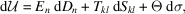where Θ is the temperature and σ is the entropy; there is summation over all dummy indices; an orthonormal frame is assumed and variance is not apparent. The mechanical energy of deformation is given by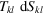(see Section 1.3.2.8). Let us consider the Gibbs free-energy functiondefined by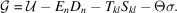Differentiation ofgives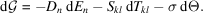The extensive parameters are therefore partial derivatives of the free energy: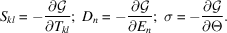Each of these quantities may be expanded by performing a further differentiation in terms of the intensive parameters,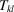,and Θ. We have, to the first order,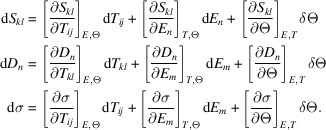To a first approximation, the partial derivatives may be considered as constants, and the above relations may be integrated: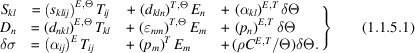This set of equations is the equivalent of relation (1.1.1.6)of Section 1.1.1.3, which gives the coefficients of the matrix of physical properties. These coefficients are:

 (i) For the principal properties: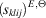: elastic compliances at constant temperature and field;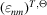: dielectric constant at constant temperatures and stress;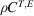: heat capacity per unit volume at constant stress and field (is the specific mass and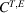is the specific heat at constant stress and field). (ii) For the other properties: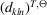and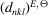are the components of the piezoelectric effect and of the converse effect. They are represented by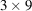and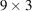matrices, respectively. One may notice that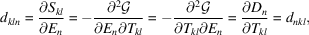which shows again that the components of two properties that are symmetric with respect to the leading diagonal of the matrix of physical properties are equal (Section 1.1.1.4) and that the corresponding matrices are transpose to one another.

In a similar way,

 (a) the matrices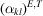of the thermal expansion and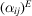of the piezocalorific effect are transpose to one another; (b) the components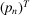of the pyroelectric and of the electrocalorific effects are equal.

Remark. The piezoelectric effect, namely the existence of an electric polarization P under an applied stress, is always measured at zero applied electric field and at constant temperature. The second equation of (1.1.5.1)becomes under these circumstances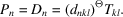Remark. Equations (1.1.5.1)are, as has been said, first-order approximations because we have assumed the partial derivatives to be constants. Actually, this approximation is not correct, and in many cases it is necessary to take into account the higher-order terms as, for instance, in:

 (a) nonlinear elasticity (see Sections 1.3.6and 1.3.7); (b) electrostriction; (c) nonlinear optics (see Chapter 1.7); (d) electro-optic and piezo-optic effects (see Sections 1.6.6and 1.6.7).

#### 1.1.5.2. Other forms of the piezoelectric constants

| top | pdf |

We use here another Gibbs function, the electric Gibbs function,, defined by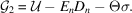Differentiation ofgives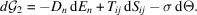It follows that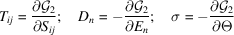and a set of relations analogous to (1.1.5.1):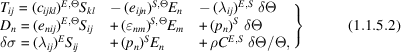where the components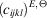are the isothermal elastic stiffnesses at constant field and constant temperature,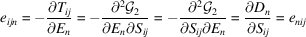are the piezoelectric stress coefficients at constant strain and constant temperature,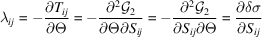are the temperature-stress constants and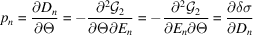are the components of the pyroelectric effect at constant strain.

The relations between these coefficients and the usual coefficients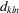are easily obtained:

 (i) At constant temperature and strain: if one puts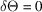and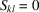in the first equation of (1.1.5.1)and (1.1.5.2), one obtains, respectively,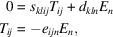from which it follows that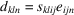at constant temperature and strain. (ii) At constant temperature and stress: if one putsand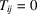, one obtains in a similar way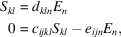from which it follows that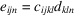at constant temperature and stress.

#### 1.1.5.3. Relation between the pyroelectric coefficients at constant stress and at constant strain

| top | pdf |

By combining relations (1.1.5.1)and (1.1.5.2), it is possible to obtain relations between the pyroelectric coefficients at constant stress,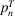, and the pyroelectric coefficients at constant strain,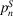, also called real pyroelectric coefficients,. Let us putand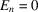in the first equation of (1.1.5.1). For a given variation of temperature,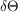, the observed strain is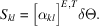From the second equations of (1.1.5.1)and (1.1.5.2), it follows that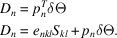Substituting the expression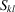and eliminating, it follows that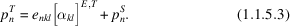This relation shows that part of the pyroelectric effect is actually due to the piezoelectric effect.

#### 1.1.5.4. Adiabatic study

| top | pdf |

Piezoelectric resonators usually operate at a high frequency where there are no heat exchanges, and therefore in an adiabatic regime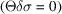. From the third equation of (1.1.5.1), we obtain a relation between the temperature variation, the applied stress and the electric field: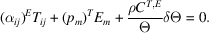If we substitute this relation in the two other relations of (1.1.5.1), we obtain two equivalent relations, but in the adiabatic regime: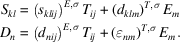By comparing these expressions with (1.1.5.1), we obtain the following relations between the adiabatic and the isothermal coefficients: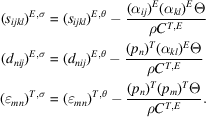### References

Mason, W. P. (1966). Crystal physics of interaction processes. London: Academic Press.
Nye, J. F. (1985). Physical properties of cystals, revised ed. Oxford University Press.
Sirotin, Y. I. & Shaskol'skaya, M. P. (1982). Fundamentals of crystal physics. Moscow: Mir.